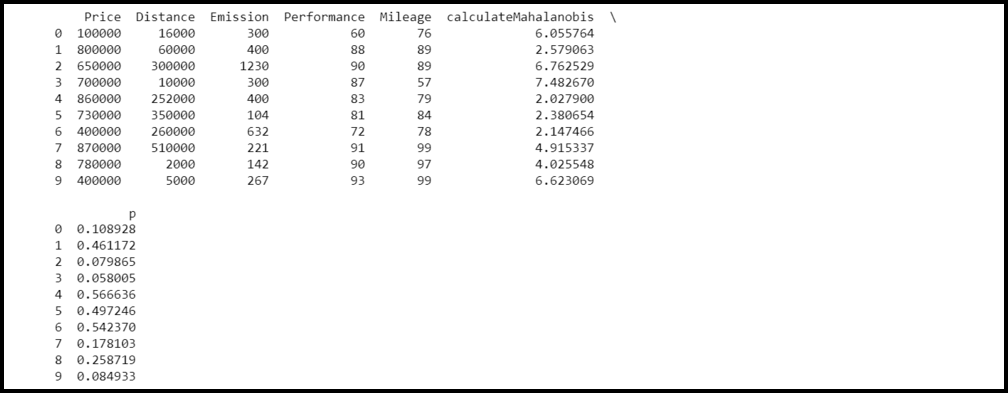# How To Calculate Mahalanobis Distance in Python

• Difficulty Level : Easy
• Last Updated : 21 Feb, 2022

Mahalanobis distance is defined as the distance between two given points provided that they are in multivariate space. This distance is used to determine statistical analysis that contains a bunch of variables.

The user needs to install and import the following libraries for calculating Mahalanobis Distance in Python:

• numpy
• pandas
• scipy

Syntax to install all the above packages:

`pip3 install numpy pandas scipy`

Step 1: The first step is to import all the libraries installed above.

## Python3

 `# Importing libraries`` ` `import` `numpy as np``import` `pandas as pd ``import` `scipy as stats`

Step 2: Creating a dataset. Consider a data of 10 cars of different brands. The data has five sections:

• Price
• Distance
• Emission generated
• Performance
• Mileage

## Python3

 `# data ``data ``=` `{ ``'Price'``: [``100000``, ``800000``, ``650000``, ``700000``,``                   ``860000``, ``730000``, ``400000``, ``870000``,``                   ``780000``, ``400000``],``         ``'Distance'``: [``16000``, ``60000``, ``300000``, ``10000``,``                      ``252000``, ``350000``, ``260000``, ``510000``,``                      ``2000``, ``5000``],``         ``'Emission'``: [``300``, ``400``, ``1230``, ``300``, ``400``, ``104``,``                      ``632``, ``221``, ``142``, ``267``],``         ``'Performance'``: [``60``, ``88``, ``90``, ``87``, ``83``, ``81``, ``72``, ``                         ``91``, ``90``, ``93``],``         ``'Mileage'``: [``76``, ``89``, ``89``, ``57``, ``79``, ``84``, ``78``, ``99``, ``                     ``97``, ``99``]``           ``}`` ` `# Creating dataset``df ``=` `pd.DataFrame(data,columns``=``[``'Price'``, ``'Distance'``,``                                ``'Emission'``,``'Performance'``,``                                ``'Mileage'``])`

Step 3: Determining the Mahalanobis distance for each observation.

## Python3

 `# Importing libraries`` ` `import` `numpy as np``import` `pandas as pd ``import` `scipy as stats`` ` `# calculateMahalanobis function to calculate``# the Mahalanobis distance``def` `calculateMahalanobis(y``=``None``, data``=``None``, cov``=``None``):`` ` `    ``y_mu ``=` `y ``-` `np.mean(data)``    ``if` `not` `cov:``        ``cov ``=` `np.cov(data.values.T)``    ``inv_covmat ``=` `np.linalg.inv(cov)``    ``left ``=` `np.dot(y_mu, inv_covmat)``    ``mahal ``=` `np.dot(left, y_mu.T)``    ``return` `mahal.diagonal()`` ` `# create new column in dataframe that contains ``# Mahalanobis distance for each row``df[``'calculateMahalanobis'``] ``=` `mahalanobis(x``=``df, data``=``df[[``'Price'``, ``'Distance'``,``                                                        ``'Emission'``,``'Performance'``,``                                                        ``'Mileage'``]])`

Combining all steps:

Example:

## Python3

 `# Importing libraries`` ` `import` `numpy as np``import` `pandas as pd``import` `scipy as stats`` ` `# calculateMahalanobis function to calculate``# the Mahalanobis distance``def` `calculateMahalanobis(y``=``None``, data``=``None``, cov``=``None``):`` ` `    ``y_mu ``=` `y ``-` `np.mean(data)``    ``if` `not` `cov:``        ``cov ``=` `np.cov(data.values.T)``    ``inv_covmat ``=` `np.linalg.inv(cov)``    ``left ``=` `np.dot(y_mu, inv_covmat)``    ``mahal ``=` `np.dot(left, y_mu.T)``    ``return` `mahal.diagonal()`` ` `# data``data ``=` `{ ``'Price'``: [``100000``, ``800000``, ``650000``, ``700000``, ``                   ``860000``, ``730000``, ``400000``, ``870000``,``                   ``780000``, ``400000``],``         ``'Distance'``: [``16000``, ``60000``, ``300000``, ``10000``, ``                      ``252000``, ``350000``, ``260000``, ``510000``, ``                      ``2000``, ``5000``],``         ``'Emission'``: [``300``, ``400``, ``1230``, ``300``, ``400``, ``104``,``                      ``632``, ``221``, ``142``, ``267``],``         ``'Performance'``: [``60``, ``88``, ``90``, ``87``, ``83``, ``81``, ``72``, ``                         ``91``, ``90``, ``93``],``         ``'Mileage'``: [``76``, ``89``, ``89``, ``57``, ``79``, ``84``, ``78``, ``99``, ``                     ``97``, ``99``]``           ``}`` ` `# Creating dataset``df ``=` `pd.DataFrame(data,columns``=``[``'Price'``, ``'Distance'``,``                                ``'Emission'``,``'Performance'``, ``                                ``'Mileage'``])`` ` `# Creating a new column in the dataframe that holds``# the Mahalanobis distance for each row``df[``'calculateMahalanobis'``] ``=` `calculateMahalanobis(y``=``df, data``=``df[[``  ``'Price'``, ``'Distance'``, ``'Emission'``,``'Performance'``, ``'Mileage'``]])`` ` `# Display the dataframe``print``(df)`

Output:## Computing the p-value for every Mahalanobis distance

Now let us compute the p-value for every Mahalanobis distance of each observation of the dataset. As you from the above output, some of the Mahalanobis distances are significantly larger than other values. To compute whether some of the distances are statistically significant we need to find their p-value. The p-value for each of the distances is the same as the p-value that belongs to the Chi-Square statistic of the Mahalanobis distance having degrees of freedom equal to k-1, where k = number of variables. So, in this case, we’ll use a degree of freedom of 5-1 = 4.

Example:

## Python3

 `# Importing libraries`` ` `import` `numpy as np``import` `pandas as pd``import` `scipy as stats``from` `scipy.stats ``import` `chi2`` ` `# calculateMahalanobis Function to calculate``# the Mahalanobis distance``def` `calculateMahalanobis(y``=``None``, data``=``None``, cov``=``None``):`` ` `    ``y_mu ``=` `y ``-` `np.mean(data)``    ``if` `not` `cov:``        ``cov ``=` `np.cov(data.values.T)``    ``inv_covmat ``=` `np.linalg.inv(cov)``    ``left ``=` `np.dot(y_mu, inv_covmat)``    ``mahal ``=` `np.dot(left, y_mu.T)``    ``return` `mahal.diagonal()`` ` `# data``data ``=` `{ ``'Price'``: [``100000``, ``800000``, ``650000``, ``700000``,``                   ``860000``, ``730000``, ``400000``, ``870000``,``                   ``780000``, ``400000``],``         ``'Distance'``: [``16000``, ``60000``, ``300000``, ``10000``, ``                      ``252000``, ``350000``, ``260000``, ``510000``,``                      ``2000``, ``5000``],``         ``'Emission'``: [``300``, ``400``, ``1230``, ``300``, ``400``, ``104``,``                      ``632``, ``221``, ``142``, ``267``],``         ``'Performance'``: [``60``, ``88``, ``90``, ``87``, ``83``, ``81``, ``72``,``                         ``91``, ``90``, ``93``],``         ``'Mileage'``: [``76``, ``89``, ``89``, ``57``, ``79``, ``84``, ``78``, ``99``,``                     ``97``, ``99``]``           ``}`` ` `# Creating dataset``df ``=` `pd.DataFrame(data,columns``=``[``'Price'``, ``'Distance'``,``                                ``'Emission'``,``'Performance'``,``                                ``'Mileage'``])`` ` `# Creating a new column in the dataframe that holds``# the Mahalanobis distance for each row``df[``'Mahalanobis'``] ``=` `calculateMahalanobis(y``=``df, data``=``df[[``  ``'Price'``, ``'Distance'``, ``'Emission'``,``'Performance'``, ``'Mileage'``]])`` ` `# calculate p-value for each mahalanobis distance``df[``'p'``] ``=` `1` `-` `chi2.cdf(df[``'Mahalanobis'``], ``3``)`` ` `# display first five rows of dataframe``print``(df)`

Output:Interpretation:

Generally, the observation having a p-value less than 0.001 is assumed to be an outlier. In this example, there is no outlier as all the p-values are greater than 0.001.

My Personal Notes arrow_drop_up